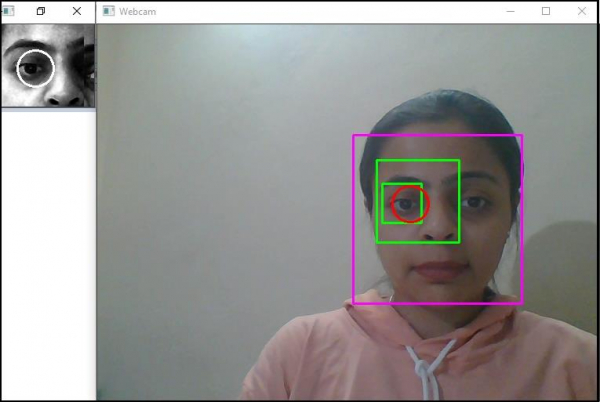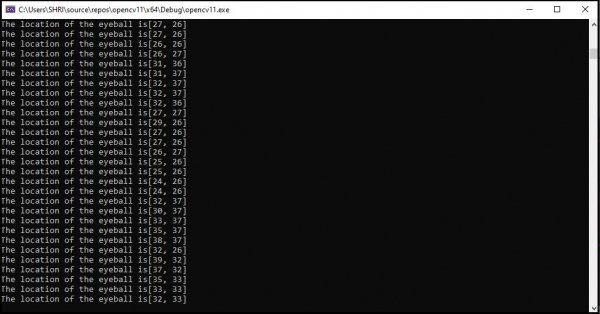# How to detect and track the motion of eyeball in OpenCV using C++?

Here, we will learn how to detect and track the motion of eyeball in OpenCV.

The following program demonstrates to detect the eyeball and track the location.

## Example

#include<iostream>
#include<opencv2/core/core.hpp>
#include<opencv2/highgui/highgui.hpp>
#include<opencv2/imgproc/imgproc.hpp>
#include<opencv2/objdetect/objdetect.hpp>
#include<string>
using namespace cv;
using namespace std;
Vec3f eyeBallDetection(Mat& eye, vector<Vec3f>& circles) {
vector<int>sums(circles.size(), 0);
for (int y = 0; y < eye.rows; y++) {
uchar* data = eye.ptr<uchar>(y);
for (int x = 0; x < eye.cols; x++) {
int pixel_value = static_cast<int>(*data);
for (int i = 0; i < circles.size(); i++) {
Point center((int)round(circles[i]), (int)round(circles[i]));
if (pow(x - center.x, 2) + pow(y - center.y, 2) < pow(radius, 2)) {
sums[i] = sums[i] + pixel_value;
}
}
++data;
}
}
int smallestSum = 9999999;
int smallestSumIndex = -1;
for (int i = 0; i < circles.size(); i++) {
if (sums[i] < smallestSum) {
smallestSum = sums[i];
smallestSumIndex = i;
}
}
return circles[smallestSumIndex];
}
Rect detectLeftEye(vector& eyes) {
int leftEye = 99999999;
int index;
for (int i = 0; i < eyes.size(); i++) {
if (eyes[i].tl().x < leftEye) {
leftEye = eyes[i].tl().x;
index = i;
}
}
return eyes[index];
}
vector<Point>centers;
Point track_Eyeball;
Point makeStable(vector<Point>& points, int iteration) {
float sum_of_X = 0;
float sum_of_Y = 0;
int count = 0;
int j = max(0, (int)(points.size() - iteration));
int number_of_points = points.size();
for (j; j < number_of_points; j++) {
sum_of_X = sum_of_X + points[j].x;
sum_of_Y = sum_of_Y + points[j].y;
++count;
}
if (count > 0) {
sum_of_X /= count;
sum_of_Y /= count;
}
return Point(sum_of_X, sum_of_Y);
}
Mat grayImage;
cvtColor(frame, grayImage, COLOR_BGR2GRAY);
equalizeHist(grayImage, grayImage);
//Detecting the face//
Mat inputImage = grayImage;
vector<Rect>storedFaces;
float scaleFactor = 1.1;
int minimumNeighbour = 2;
Size minImageSize = Size(150, 150);
inputImage, storedFaces, scaleFactor, minimumNeighbour, 0 | CASCADE_SCALE_IMAGE, minImageSize);
if (storedFaces.size() == 0)return;
Mat face = grayImage(storedFaces);
//Drawing rectangle around the detected face//
int x = storedFaces.x;
int y = storedFaces.y;
int h = y + storedFaces.height;
int w = x + storedFaces.width;
rectangle(frame, Point(x, y), Point(w, h), Scalar(255, 0, 255), 2, 8, 0);
//Detecting the eyes//
Mat faceRegion = face;
vector<Rect>eyes;
float eyeScaleFactor = 1.1;
int eyeMinimumNeighbour = 2;
Size eyeMinImageSize = Size(30, 30);
if (eyes.size() != 2)return;
//Drawing the rectangle around the eyes//
for (Rect& eye : eyes) {
rectangle(frame, storedFaces.tl() + eye.tl(), storedFaces.tl() + eye.br(), Scalar(0, 255, 0), 2);
}
//Getting the left eye//
Rect eyeRect = detectLeftEye(eyes);
Mat eye = face(eyeRect);
equalizeHist(eye, eye);
//Applying Hough Circles to detect the circles in eye region//
Mat hough_Circle_Input = eye;
vector<Vec3f>circles;
int method = 3;
int detect_Pixel = 1;
int minimum_Distance = eye.cols / 8;
int threshold = 250;
int minimum_Area = 15;
int minimum_Radius = eye.rows / 8;
int maximum_Radius = eye.rows / 3;
HoughCircles(hough_Circle_Input, circles,
//Detecting the drawing circle that encloses the eyeball//
if (circles.size() > 0) {
Vec3f eyeball = eyeBallDetection(eye, circles);
Point center(eyeball, eyeball);
centers.push_back(center);
center = makeStable(centers, 5);
track_Eyeball = center;
circle(frame, storedFaces.tl() + eyeRect.tl() + center, radius, Scalar(0, 0, 255), 2);
circle(eye, center, radius, Scalar(255, 255, 255), 2);
}
cout << "The location of the eyeball is" << track_Eyeball << endl;
imshow("Eye", eye);
}
int main() {
//Capturing camera feed and calling eyeDetection function//
VideoCapture cap(0);
Mat frame;
while (1) {
cap >> frame;
imshow("Webcam", frame);
if (waitKey(30) >= 0) break;
}
return 0;
}

## Output